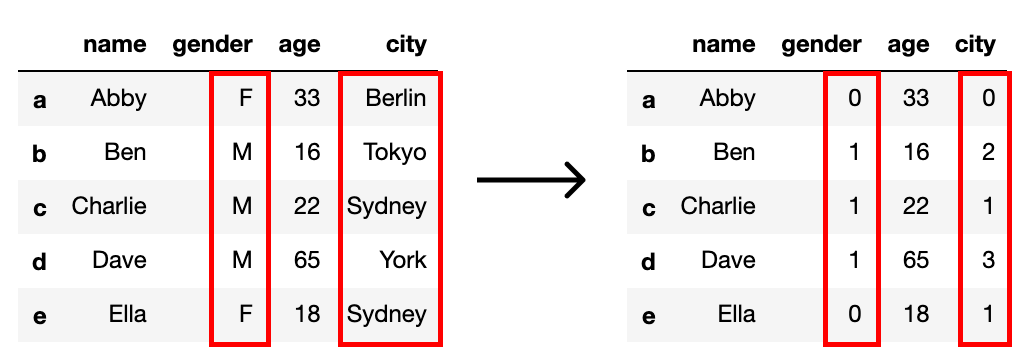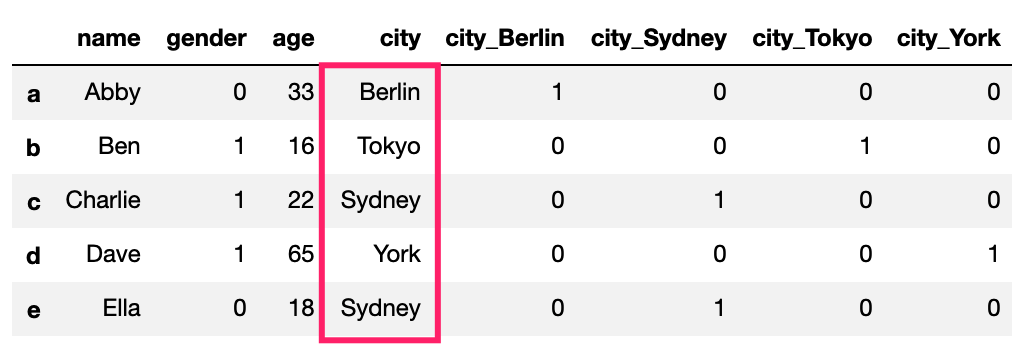Unlike Decision Tree Classifier, some machine learning models doesn't have the ability to deal with categorical data. The categorical data are often requires a certain transformation technique if we want to include them, namely Label Encoding and One-Hot Encoding.

## Label Encoding

What the Label Encoding does is transform text values to unique numeric representations. For example, 2 categorical columns "gender" and "city" were converted to numeric values, a number stands for a certain category in the column. (we didn't convert the name column because usually it will be removed when actually applying models)That's all for Label Encoding. But depending on the data, label encoding introduces a new problem. For example, we have encoded a set of city names into numerical data. But this is actually categorical data with a number indicator. Thus there is no relation between the values, i.e. in the example, York is not as 3 times as Sydney. To overcome this problem, we need to use the so called One-Hot Encoding.

## One-Hot Encoding

What the One-Hot Encoding does is, it creates dummy columns with values of 0s and 1s, depending on which column has the value. It might be easier to understand by this visualization:For illustratration purpose, I put back the original city name.

Further, we can drop one dummy column (usually the first column) since we can know the value from the rest dummy columns.

One more thing, the value type in dummy columns are object. It's better to convert them into float before applying models.

## Code

### Label Encoding

We can use the LabelEncoder() in sklearn's preprocessing.

import pandas as pd
from sklearn import preprocessing

# call LabelEncoder()
le = preprocessing.LabelEncoder()

# create a df
data = [['Abby','F',33,'Berlin'],
['Ben','M',16,'Tokyo'],
['Charlie','M',22,'Sydney'],
['Dave','M','65','York'],
['Ella','F',18,'Sydney']]
index = ['a','b','c','d','e']
columns=['name','gender','age','city']
df = pd.DataFrame(data=data, index=index, columns=columns)
df

# label encode gender and city
df['gender'] = le.fit_transform(df['gender'])
df['city'] = le.fit_transform(df['city'])
df


### One-Hot Encoding

To implement the Label Encoding and One-Hot Encoding together, we can use the get_cummies() function in Pandas:

import pandas as pd

# create a df
df = pd.DataFrame(['A','B','C','A','D'],columns=['User'])

# create dummy columns and drop the first dummy column
df_dropped = pd.get_dummies(df['User'], prefix='User', drop_first=True)

# change the data type to float
df_dropped = df_dropped.astype('float')


Here is a function I wrote to creat dummy columns based on one column in a dataframe, original and first dropped, then join the dummy columns back to the original dataframe:

# name: dum_col()
# function: creat dummy columns based on one column in a dataframe
# original and first dropped
# then join the dummy columns back to the original dataframe.
# dependencies:
#     import pandas as pd
#     import copy
# arguments:
#     df: dataframe
#     col_name: 'column_name'
#     prefix: dummy column names prefix
# by: Yuzhang Huang @ 2019.07.27
# ===============================================================
def dum_col(df,col_name,prefix):
df_new = copy.deepcopy(df) # copy a new df
df_temp = pd.get_dummies(df_new[col_name],prefix=prefix,drop_first=True) # get dummy columns
df_new = df_new.drop(columns=[col_name]) # drop original column
df_new = pd.concat([df_new, df_temp], axis=1) # join the dummy columns back to original dataframe
return df_new
`

The code of this post can be found at: